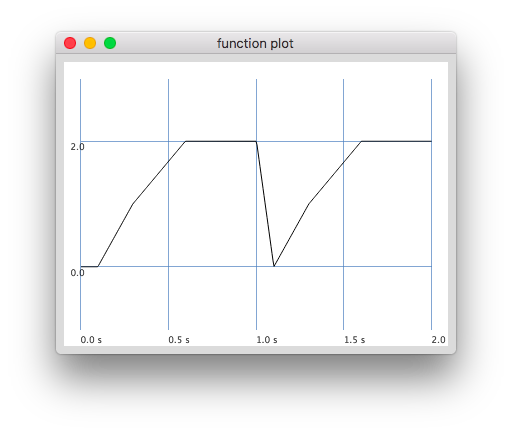# How to trigger an enveloppe that will go from 0 to 1 and stay at 1 until next trigger

Hello !

How do I make an envelope that will go from 0 to 1 in 100ms and will stay at 1 until the next trigger ?

My code is as follows :

``````(
{
var trig, tempo, drone, note;
note = 41; // fa
tempo = 70/60;

trig = TDuty.kr(Dseq([Dwrand([1/3, 2/3], [0.9, 0.1], 1), 1/3, 1/3, 1],inf) / tempo +Dwhite(-0.01, 0.01,inf));

drone = Splay.ar( (1..10).collect({ |i|
Saw.ar(
freq: note.midicps * i,
mul: 1/i
)
}));

drone = drone * EnvGen.ar(
envelope: Env.asr(0.1, 1.0, 0.1), // this is obviously not the right enveloppe
gate: trig
);

drone
}.play
)
``````

Any clue ?

Geoffroy

Hi,

have a look at the releaseNode / loopNode examples of the Env helpfile, there are quite tricky cases

http://doc.sccode.org/Classes/Env.html#examples

``````(
// you need a start segment, the loop will begin at the target of the first segment
e = Env.new([0, 0, 1], [0.1, 0.1] * 0.01, 'lin', nil, 1);
e.plot;
{ EnvGen.ar(e, Impulse.ar(100)) }.plot(0.1);
)

// due to the delay of the Env at start you'd need to
// introduce a delay with the frequency sequence (if you need one at all)

// wrap freq with Clip as Saw with 0 produces a bump

(
{
var trig, tempo, drone, note, att = 0.1, rel = 0.1;
tempo = 70/60;

trig = TDuty.ar(
Dseq([Dwrand([1/3, 2/3], [0.9, 0.1], 1), 1/3, 1/3, 1],inf) / tempo +
Dwhite(-0.01, 0.01,inf)
);
note = Demand.ar(trig, 0, Dwhite(60, 90));

drone = Splay.ar((1..10).collect({ |i|
Saw.ar(
freq: Clip.ar(DelayL.ar(note.midicps * i, 0.3, rel).poll, 50, 5000),
mul: 1/i
)
}));

drone = drone * EnvGen.ar(
envelope: Env.new([0, 0, 1], [att, rel], 'lin', nil, 1),
gate: trig
);

drone
}.play
)
``````

As Delay etc. feels a bit quirky It might be worth considering PmonoArtic.

Greetings

Daniel

1 Like

Thanks a lot Daniel !

I could not understand the `releaseNode`, `loopNode` and `offset` arguments in the Env documentation.

It makes a bit more sense now.

I also found another workaround, which is to use 1 - a perc enveloppe, like

``````(
{
var trig, tempo, drone, note;
note = 41; // fa
tempo = 70/60;

trig = TDuty.kr(Dseq([Dwrand([1/3, 2/3], [0.9, 0.1], 1), 1/3, 1/3, 1],inf) / tempo +Dwhite(-0.01, 0.01,inf));

drone = Splay.ar( (1..10).collect({ |i|
Saw.ar(
freq: note.midicps * i,
mul: 1/i
)
}));

drone = drone * (1 - EnvGen.kr(
envelope: Env.perc(0.01, 0.3),
gate: trig
));

}.play
)
``````

But that was a hack and I am happy to better understand looping enveloppes now.

Thanks again !

Re-reading your solution Daniel, I’m trying to understand loop envelopes a bit more and I’m a bit confused.

Using SC 3.10.0

``````(
e = Env.new(levels: [0, 0, 1, 2], times: [0.1, 0.2, 0.3]);
{
EnvGen.kr(envelope: e, gate: Impulse.kr(1));
}.plot(duration: 2, minval: -0.1, maxval: 2.1);
)
``````

gives :and specifying another `loopNode` does change anything.

``````(
e = Env.new(levels: [0, 0, 1, 2], times: [0.1, 0.2, 0.3], loopNode: 2);
{
EnvGen.kr(envelope: e, gate: Impulse.kr(1));
}.plot(duration: 2, minval: -0.1, maxval: 2.1);
)
``````

bringsDoes it mean `loopNode` only works with a set `releaseNode` ?

Thanks !

You’re right.
Although my example works as it is it doesn’t use the loopNode feature.
When using the loopNode it also needs a different kind of trigger definition,
in this case looping is done without trigger, only release is triggered, but in
the opposite way, by setting gate to 0.

``````(
e = Env.new(levels: [0, 1, 2, 0], times: [0.1, 0.2, 0.5], loopNode: 0, releaseNode: 2);
e.plot;
{
EnvGen.ar(envelope: e, gate: Trig.ar(1, 1.2), doneAction: 2);
}.plot(duration: 2.5, minval: -0.1, maxval: 2.1);
)``````

Thanks a lot Daniel !

It’s a bit weird that the releaseNode is actually the node at levels (value = 2) where the loopNode is the node at levels[0+1] (value = 1).

I tried another example and this is the case

``````(
e = Env.new(levels: [0, 1, 2, 3, 4, 0], times: [0.1, 0.2, 0.1, 0.1, 0.5], loopNode: 1, releaseNode: 4);
e.plot;
{
EnvGen.ar(envelope: e, gate: Trig.ar(1, 2.05), doneAction: 2);
}.plot(duration: 3, minval: -0.1, maxval: 4.1);
)
``````

It’s definitely looping back at node levels[loopNode+1] (value = 2)

Not a big deal, it works